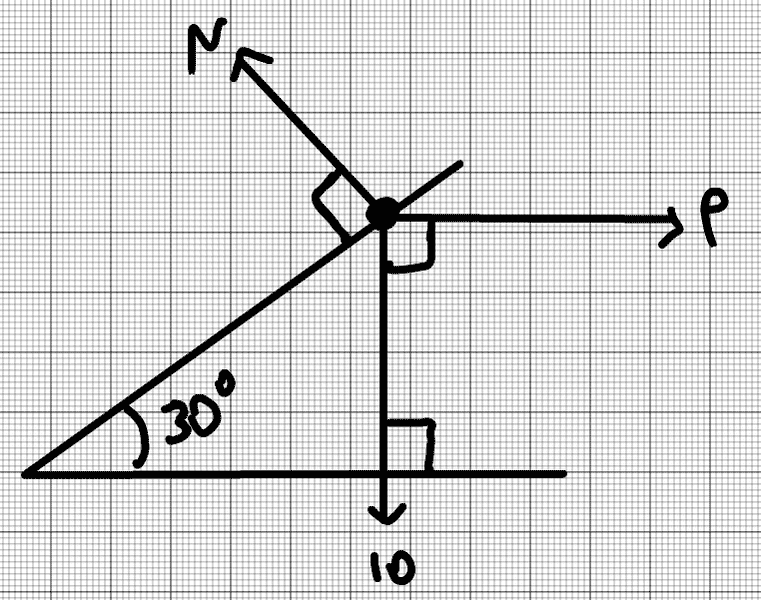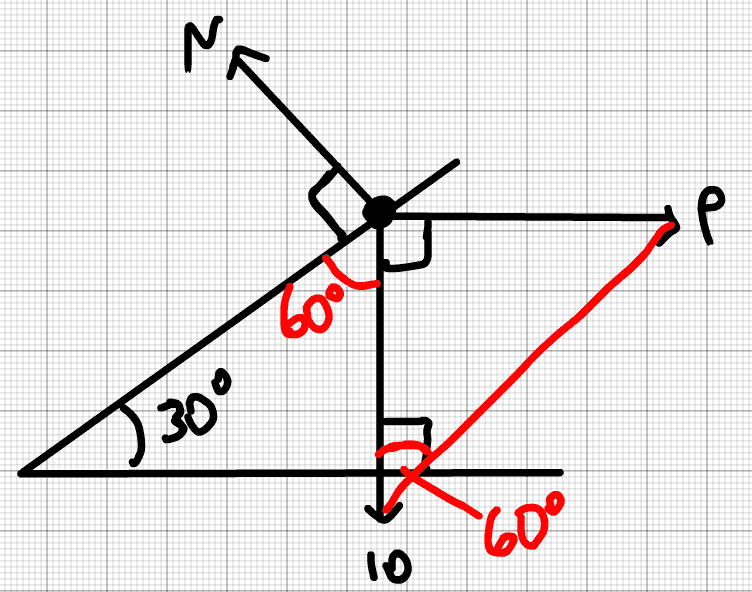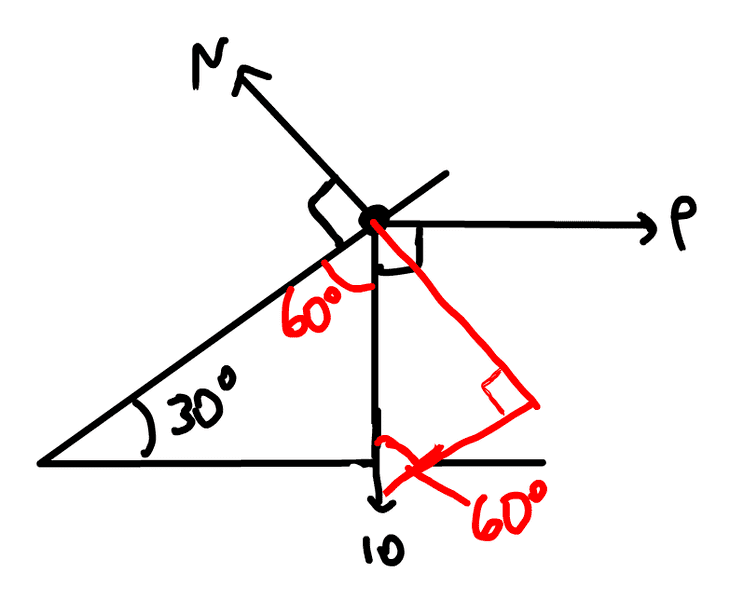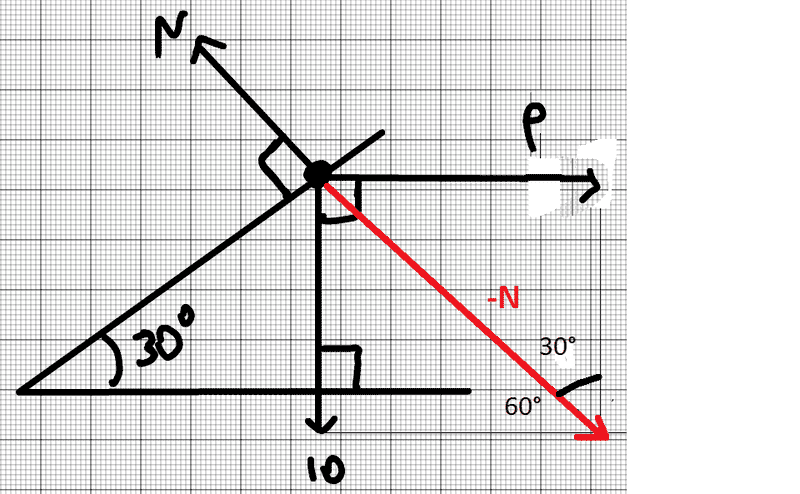# Resolving forces in equilibrium on inclined plane

## Homework Statement

Solve for the forces P and N acting on an object in equilibrium on an inclined plane.
Weight=10N, angle is 30 degrees
http://blob:https%3A//www.physicsforums.com/d431a152-5681-411b-ba57-809320539165## Homework Equations

Trigonometry, i.e. sin, cos, tan rules. The 'Z' rule (amongst others) to determine angles.

## The Attempt at a Solution

I have attempted the problem (as done with similar problems, which were correct) however the answers seem to assume that the angle in solving P is 30.
I have used in the Parallel P=10tan60=17.32N. However the answer is given by 10tan30=5.77N. Is the answer wrong? Or have I made inaccurate assumptions?In the perpendicular, N=10sin60=8.66N, however this is wrong also! The answer is given by 10/sin60Thankyou for any help.

Assume a frictionless plane.

Resolve N into X and Y directions and it will become clear.

•Issy04
Assume a frictionless plane.

Resolve N into X and Y directions and it will become clear.
The Y component of N is used to support 10N object from moving down vertically. Since we resolve N into components, then there must also be an X component.
Once you resolved N into components, N does not exist anymore but 4 forces acting on the object.

Last edited:
•Issy04
The Y component of N is used to support 10N object from moving down vertically. Since we resolve N into components, then there must also be an X component.
Once you resolved N into components, N does not exist anymore but 4 forces acting on the object.

Thank you. I have calculated the correct value of P now but I am still confused as to why N does not equal 10sin60, as P has no vertical component?

ehild
Homework Helper
The resultant of the 10 N force and P must be equal and opposite to N.#### Attachments

•Issy04
Thank you. I have calculated the correct value of P now but I am still confused as to why N does not equal 10sin60, as P has no vertical component?
Now we try to solve using triangular method since net forces equal to zero.
As you you know you can place the vector, the arrow anywhere, parallel and pointing to same direction.
10N is the base, P is opposite, and N is hypothenus.

•Issy04
Now we try to solve using triangular method since net forces equal to zero.
As you you know you can place the vector, the arrow anywhere, parallel and pointing to same direction.
10N is the base, P is opposite, and N is hypothenus.
Thank you very much! It is clear now.
The resultant of the 10 N force and P must be equal and opposite to N.

View attachment 92220